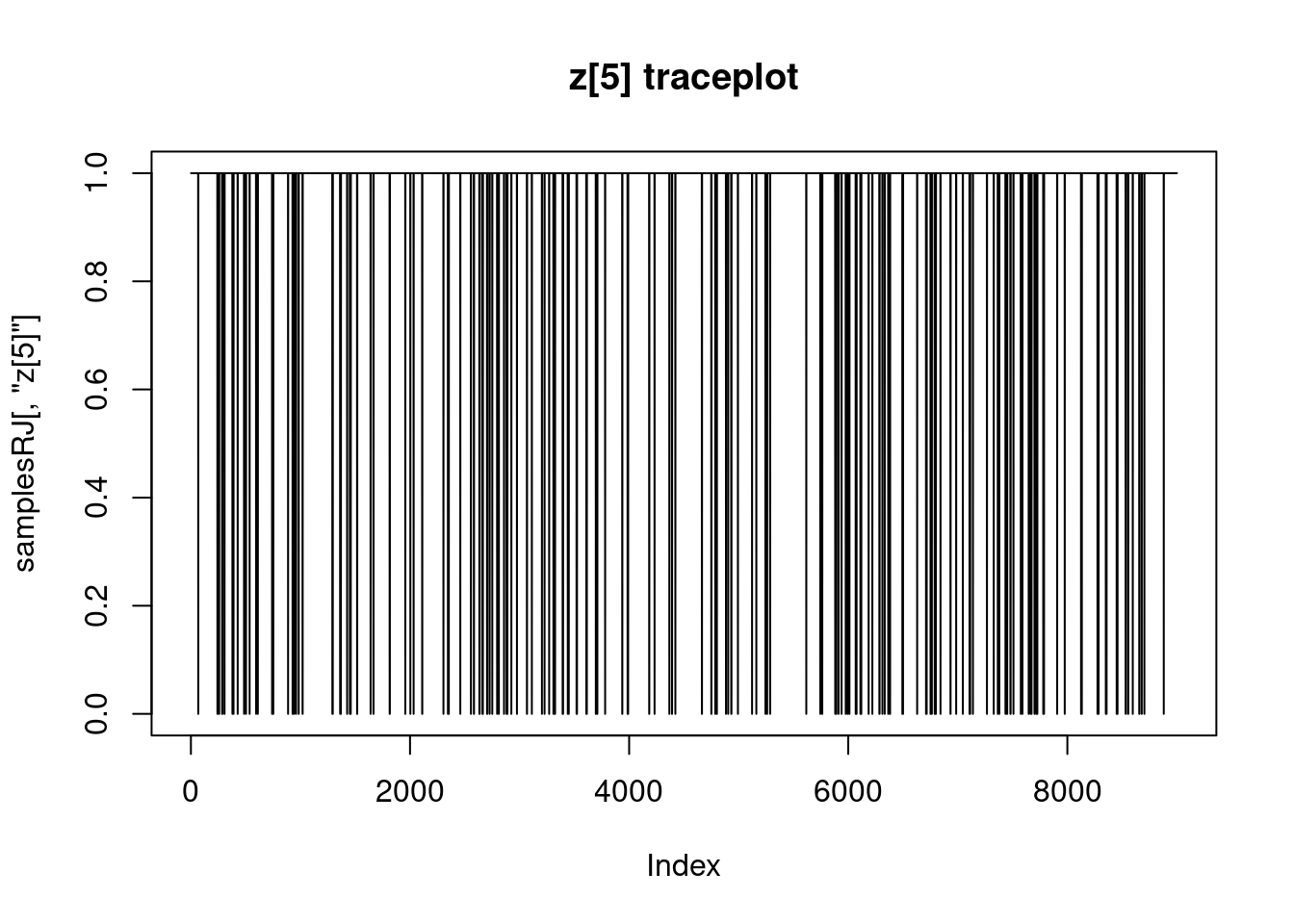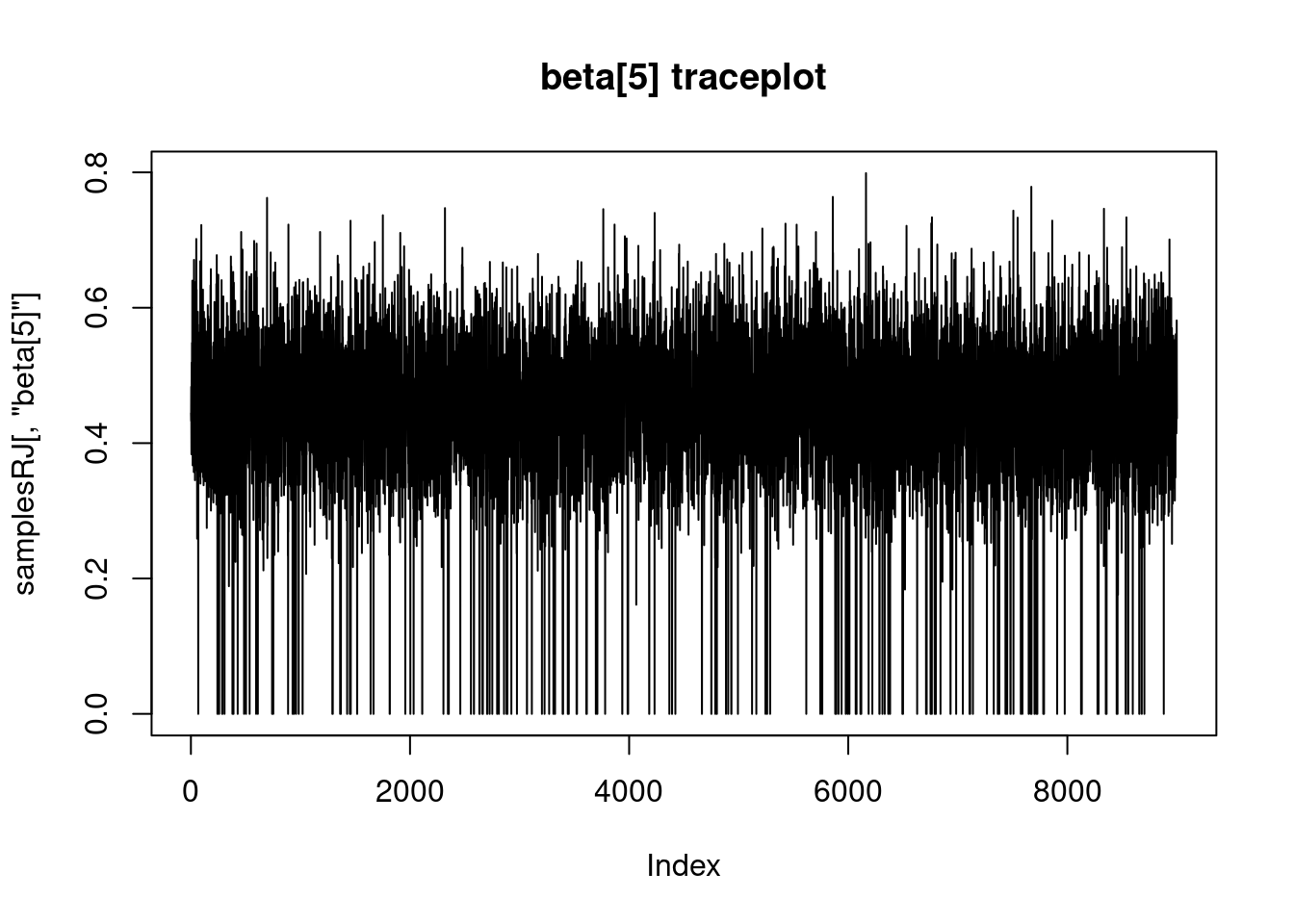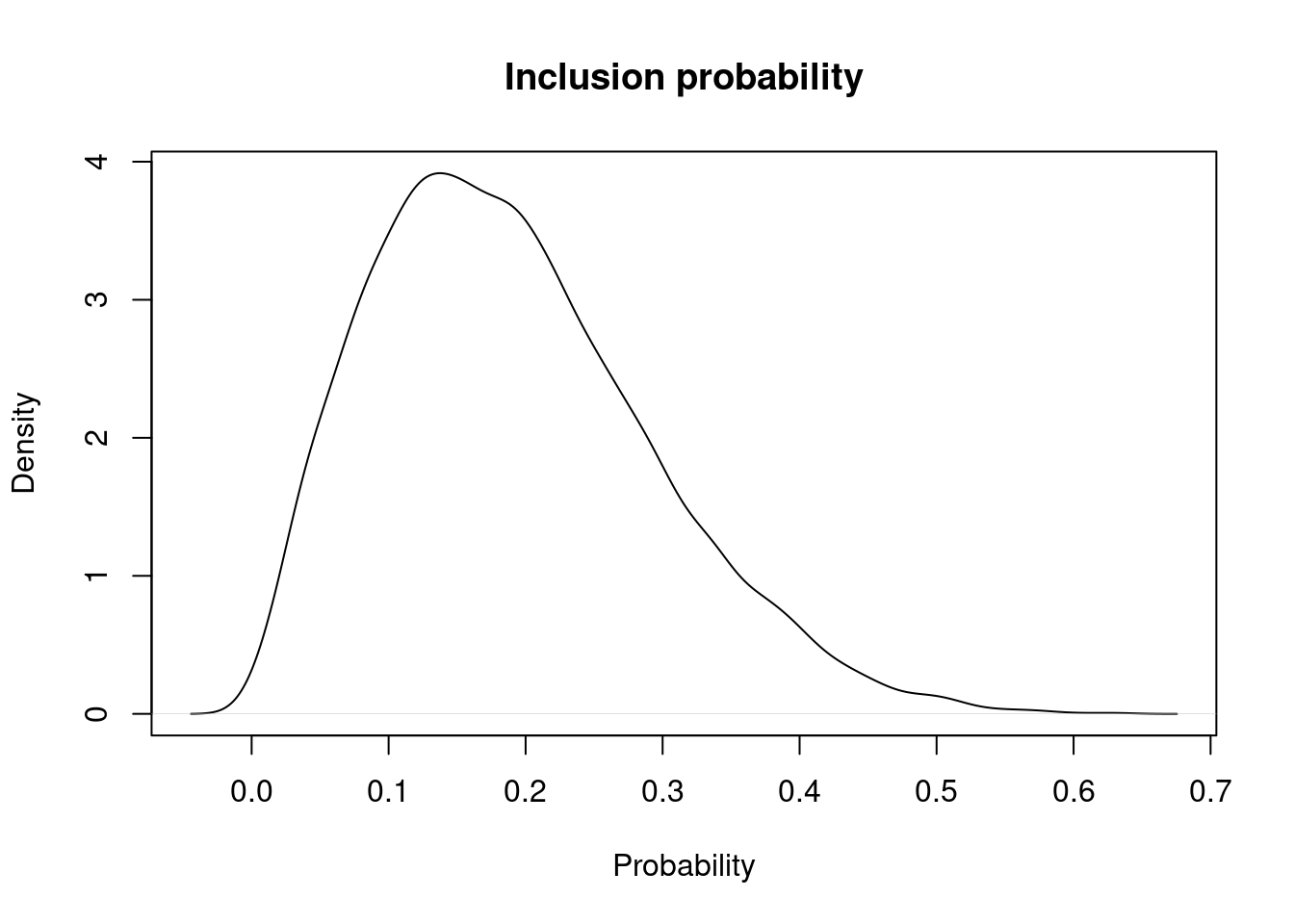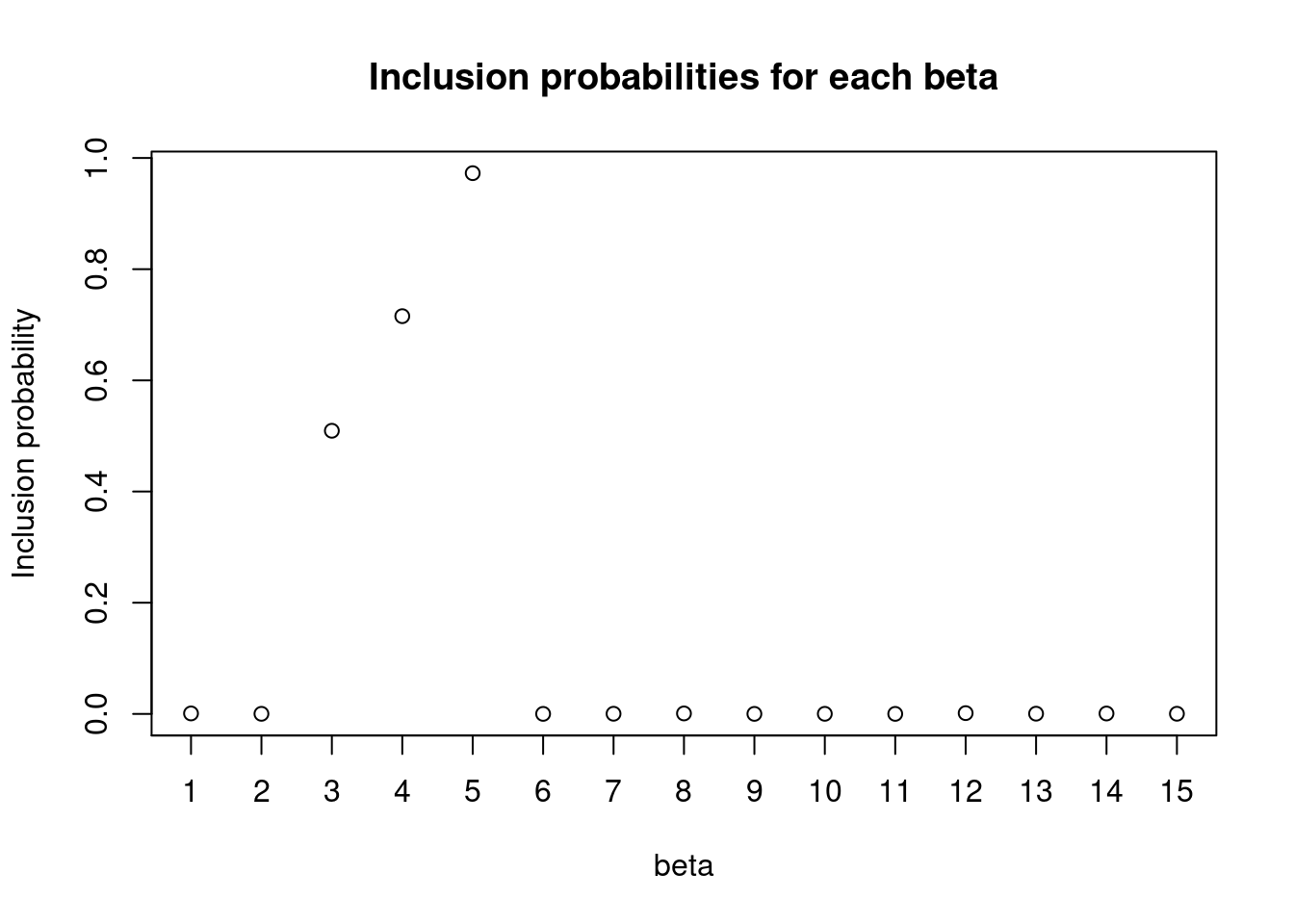Reversible Jump MCMC (RJMCMC) is a method for sampling across models with a different numbers of dimensions, which finds natural application in Bayesian variable selection problems. In this setting, RJMCMC requires a proposal distribution for the coefficient of a variable when the variable is proposed to be included in the model. NIMBLE uses a univariate normal proposal distribution.

There are two ways to use RJMCMC variable selection in your model. If you know the prior probability for inclusion of a variable in the model, you can use that directly in RJMCMC without modifying your model. If you need the prior probability for inclusion in the model to be a model node itself, such as if it will have a prior and be estimated, you will need to write the model with indicator variables. The latter is more flexible and is illustrated in the example below.

More information can be found in the user manual and via help(configureRJ).

## Linear regression example

For simplicity we consider a linear regression example with 15 explanatory variables, of which five have real effects. The other 10 have null effects, i.e. the corresponding coefficient is equal to zero.

library(nimble, warn.conflicts = FALSE)
## nimble version 1.0.1 is loaded.
##
## Note for advanced users who have written their own MCMC samplers:
##   As of version 0.13.0, NIMBLE's protocol for handling posterior
##   predictive nodes has changed in a way that could affect user-defined
##   samplers in some situations. Please see Section 15.5.1 of the User Manual.
lmCode <- nimbleCode({
sigma ~ dunif(0, 20)
psi ~ dunif(0,1)   ## prior on inclusion probability

for(i in 1:numVars) {
z[i] ~ dbern(psi) ## indicator variable for each coefficient
beta[i] ~ dnorm(0, sd = 100)
zbeta[i] <- z[i] * beta[i]  ## indicator * beta
}
for(i in 1:n) {
pred.y[i] <- inprod(X[i, 1:numVars], zbeta[1:numVars])
y[i] ~ dnorm(pred.y[i], sd = sigma)
}
})
## Generate data and define model constants
set.seed(1)
numVars = 15
n = 100
## Simulate explanatory variables
X <- matrix(rnorm(n*numVars), nrow = n, ncol = numVars)
lmConstants <- list(numVars = numVars, n = n, X = X)
lmModel <- nimbleModel(lmCode, constants = lmConstants)
## Defining model
## Building model
## Setting data and initial values
## Running calculate on model
##   [Note] Any error reports that follow may simply reflect missing values in model variables.
## Checking model sizes and dimensions
##   [Note] This model is not fully initialized. This is not an error.
##          To see which variables are not initialized, use model$initializeInfo(). ## For more information on model initialization, see help(modelInitialization). We can simulate data using the nimbleModel we just created by initializing the model parameters and calling the simulate() method. ## Set the first give coefficients to be non-zero and the remaining ## ones to be zero. true_betas <- c(c(0.1, 0.2, 0.3, 0.4, 0.5), rep(0, 10)) lmModel$beta <- true_betas
lmModel$sigma <- 1 lmModel$z <- rep(1, 15)
lmModel$psi <- 0.5 this_will_be_NA <- lmModel$calculate() ## Update nodes that depend on values we just set..
set.seed(0) ## Make this example reproducible
lmModel$simulate('y') ## Simulate y lmModel$calculate() ## Update calculations
##  -226.7382
lmModel$setData('y') ## Mark the simulated y values as data lmData = list(y = lmModel$y) ## Record the simulated y values for use below

## Configuring RJMCMC

The RJCMCM sampler can be added to the MCMC configuration by calling the function configureRJ(). In this example, z are indicator variables associated with the coefficients beta. We pass them to configureRJ using the arguments indicatorNodes and targetNodes. The control argument allows us to specify the mean and the scale of the normal proposal distribution.

lmConf <- configureMCMC(lmModel)
## ===== Monitors =====
## thin = 1: beta, psi, sigma
## ===== Samplers =====
## RW sampler (2)
##   - sigma
##   - psi
## conjugate sampler (15)
##   - beta[]  (15 elements)
## binary sampler (15)
##   - z[]  (15 elements)
lmConf$addMonitors('z') ## thin = 1: beta, psi, sigma, z configureRJ(lmConf, targetNodes = 'beta', indicatorNodes = 'z', control = list(mean = 0, scale = .2)) Checking the assigned samplers, we can see that indicator parameters are assigned to an RJ_indicator sampler whose targetNode is the corresponding coefficient, while beta parameters are assigned to a RJ_toggled sampler, which is used only when its target parameter is included in the model. ## Check the assigned samplers lmConf$printSamplers(c("z", "beta"))
##  RJ_toggled sampler: beta,  samplerType: conjugate_dnorm_dnorm_linear
##  RJ_indicator sampler: z,  mean: 0,  scale: 0.20000000000000001,  targetNode: beta

## Build and run the RJMCMC

mcmcRJ <- buildMCMC(lmConf)

cLmModel <- compileNimble(lmModel)
## Compiling
##   [Note] This may take a minute.
##   [Note] Use 'showCompilerOutput = TRUE' to see C++ compilation details.
CMCMCRJ <- compileNimble(mcmcRJ, project = lmModel)
## Compiling
##   [Note] This may take a minute.
##   [Note] Use 'showCompilerOutput = TRUE' to see C++ compilation details.
set.seed(100)
system.time(samplesRJ <- runMCMC(CMCMCRJ, niter = 100000, nburnin = 10000, thin = 10))
## running chain 1...
## |-------------|-------------|-------------|-------------|
## |-------------------------------------------------------|
##    user  system elapsed
##  16.969   0.008  16.978

## Look at the results

For each variable we can look at the sampled values of the indicator and corresponding coefficient.

plot(samplesRJ[,'z'], type = 'l', main = "z traceplot")plot(samplesRJ[,'beta'], type = 'l', main = "beta traceplot")### Posterior inclusion probability, psi

Here is the posterior density of inclusion probability.

plot(density(samplesRJ[,'psi']), main = "Inclusion probability", xlab = "Probability", ylab = "Density")### Individual inclusion probabilities

Here are the marginal probabilities of inclusion for each variable.

zNames <- lmModel\$expandNodeNames('z')
zCols <- which(colnames(samplesRJ) %in% zNames)
posterior_inclusion_prob <- colMeans(samplesRJ[,zCols])
plot(1:length(true_betas), posterior_inclusion_prob,
xaxt = 'none',
xlab = "beta", ylab = "Inclusion probability",
main = "Inclusion probabilities for each beta")
axis(1, seq(0,15,1))### Model probabilities

Finally we can look at the posterior probabilities for every model that was explored by the MCMC, coded by the vector of indicator variables z.

library(data.table)

binary <- as.data.table((samplesRJ[, zCols] != 0)+ 0)
res <- binary[,.N, by=names(binary)]
res <-res[order(N, decreasing = T)]
res <- res[, prob := N/dim(samplesRJ)]

res[1:5, ]
##    z z z z z z z z z z z z z z
## 1:    0    0    1    1    1    0    0    0    0     0     0     0     0     0
## 2:    0    0    0    1    1    0    0    0    0     0     0     0     0     0
## 3:    0    0    1    0    1    0    0    0    0     0     0     0     0     0
## 4:    0    0    0    0    1    0    0    0    0     0     0     0     0     0
## 5:    0    0    0    0    0    0    0    0    0     0     0     0     0     0
##    z    N       prob
## 1:     0 3305 0.36722222
## 2:     0 3044 0.33822222
## 3:     0 1222 0.13577778
## 4:     0 1130 0.12555556
## 5:     0  174 0.01933333